# KSEEB Solutions for Class 7 Maths Chapter 11 Perimeter and Area Ex 11.1

Students can Download Chapter 11 Perimeter and Area Ex 11.1, Question and Answers, Notes Pdf, KSEEB Solutions for Class 7 Maths, Karnataka State Board Solutions help you to revise complete Syllabus and score more marks in your examinations.

## Karnataka State Syllabus Class 7 Maths Chapter 11 Perimeter and Area Ex 11.1

Question 1.
The length and the breadth of a rectangular piece of land are 500 m and 300 m respectively. Find
i) its area
Solution: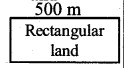Length of the land = l = 500 m
Breadth of the land = b = 300 m
∴ The Area of rectangular piece of land
l × b = 500 × 300
= 150,000 Sq. m.

ii) The cost of the. land, if 1 m2 of the land costs 10,000.
Solution:
The cost of rectangular piece of land per
1 Sq m = ₹ 10,000
∴ Total cost of that land = 150,000 × 10,000 = ₹ 1,500,000,000Question 2.
Find the area of a square park whose perimeter is 320 m.
Solution: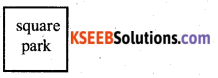Perimeter of the sq park = 320 m
∴ One side of the sq part = $$\frac{320}{4}$$ = 80 m
∴ Area of the square park = a2 = side2 = 802
80 × 80 = 6400 Sq.m

Question 3.
Find the breadth of a rectangular plot of land, if its area is 440 m2 and the length is 22 m. Also find its perimeter.
Solution:
Area of a rectangular filed = l × b = 440 sq m.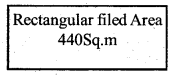Length of a rectangular field =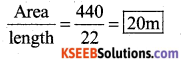perimeter of the rectangular filed = 2(l + b)
2 (20 + 22)
2(42) = 84 mQuestion 4.
The perimeter of a rectangular sheet is 100 cm. If the length is 35 cm, find its breadth. Also find the area.
Solution:
Perimeter of the rectangular sheet = 100 cm
length of the rectangular sheet = 35 cm
Breadth of the rectangular sheet = 2(l + b) = 100
= 2(35 + b)= 100
= 70 + 2b = 100
= 2b = 100 – 70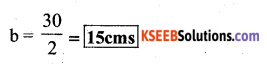∴ Area = l × b = 35 × 15 = 525 sq.cms

Question 5.
The area of a square park is the same as of a rectangular park. If the side of the square park is 60 m and the length of the rectangular park is 90 m, find the breadth of the rectangular park.
Solution: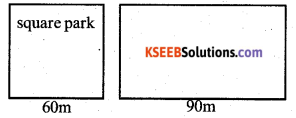Area of the sq park = Area of rectangular park
a = side = 60 m
a2 = l × b
602 = 90 × b
3600 = 90 × b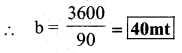∴ The breadth of a rectangular park = 40 mts.Question 6.
A wire is the shape of a rectangle. Its length is 40 cm and breadth is 22 cm. If the same wire is rebent in the shape of a square, what will be the measure of each side? Also, find which shape encloses more area?
Solution:
The shape of the wire rectangle.
The perimeter of this rectangle
Total or length of wire =2 (l + b)
length = 40 cm breadth = 22 cm
= 2(40 + 22)
= 2(62) = 124 cm.
If it is reshaped in the form of sq, its side}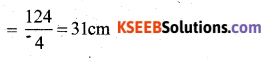∴ Its square = side × side = 31 × 31 = 961 sq. cms
Rectangle’s Area = l × b = 40 × 22 = 880 sq.cms.
The area of the square encloses more area than the area of the rectangle.

Question 7.
The perimeter of a rectangle is 130 cm. If the breadth of the rectangle 30 cm, find its length. Also find the area of the rectangle.
Solution:
p = perimeter of a rectangle = 130 cm
l = length of a rectangle = l = ?
b = breadth of a rectangle = b = 30 cm
p = 2 (l + b)
130 = 2l + 2 × 30
2l = 130 – 60 = 70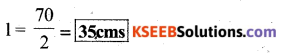∴ Area of a rectangle = l × b = 30 × 35
= 1050 sq.cmsQuestion 8.
A door of length 2 m and breadth 1 m is fitted in a wall. The length of the wall is 4.5 m and the breadth is 3.6 m (Fig 11.6). Find the cost of whitewashing the wall, if the rate of whitewashing the wall is ₹ 20 per m2
Solution: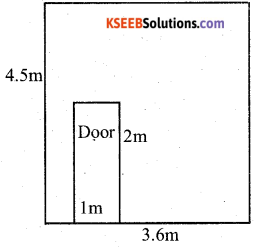Area of the wall = l × b
length of the wall = 4.5 m
the breadth of the wall = 3.6 m
Area = 4.5 × 3.6 = 16.2 sq.m
length of the door = 2 m
the breadth of the door = 1 m
Area of the door = 2 × 1 = 2 sq. m
Area of the wall excluding (without) door = 16.20 – 2 = 14.20 sq.m
Rate of whitewashing the wall = Rs. 20/ sq.m
∴ The total cost of whitewashing the wall = 14.20 × 20 = Rs. 284.00